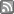# Current Research:

The main research areas in the Victoria University Gravity Group are:

## General Background

The main theme of research for the Victoria University Gravity Group, is the interface between classical gravity and quantum physics. This very broad topic is one aspect of the search for a theory of "quantum gravity", or more precisely a theory that in one limit approximately reproduces ordinary quantum physics and in another limit approximately reproduces classical Einstein gravity (general relativity).

This is not necessarily the same as quantizing Einstein gravity.

It is relatively easy to see that some sort of theory of unified theory of "quantum gravity" is necessary.

Consider an object of energy E, its de Broglie wavelength is then

λ = (hc)/(2 π E)

As long as the physical size of the object is large compared to its de Broglie wavelength, it can be adequately treated using classical physics. Once the physical size of the particle is smaller than its Compton wavelength one needs to describe the particle using quantum physics. The curve (hyperbola) described by

(size) = (h c)/(2 π energy)

is roughly speaking the border that separates the classical aspect from the quantum aspect. On the other hand, if we consider Einstein gravity, then the Schwarzschild radius of an object of energy E (and mass m = E/c²) is given by

r_{Schwarzschild} = G m/c² = G E/c^4

As long as the size of the object is large compared to its Schwarzschild radius, then it can adequately be treated using special relativity and Newton's theory of gravity. Once the physical size of the object is smaller than its Schwarzschild radius then it forms a black hole and must be treated using Einstein's theory of gravity, general relativity. Thus the curve (straight line)

(size) = G (energy)/c^4

is roughly speaking the border that separates ordinary uncollapsed matter from collapsed black holes. The everyday world of classical uncollapsed objects lies above both of these curves. The usual quantum world of particle physics and solid state physics lies below the de Broglie hyperbola, but above the Schwarzschild line. In these situations we are dealing with processes where quantum physics is important but gravity is negligible.

The black holes of interest in astrophysics and cosmology are large classical black holes, they lie above the de Broglie hyperbola but below the Schwarzschild line. Astrophysical black holes are collapsed so that gravity is certainly important, but can to an adequate approximation be treated classically, so that classical general relativity is sufficient to describe most of the relevant physical processes.

But there is a fourth region of the diagram that lies below both curves, this is the realm of the quantum black hole. These are objects that lie below both the de Broglie hyperbola and the Schwarzschild line, they are both quantum mechanical and gravitationally collapsed, and to study these quantum black hole we will need some sort of theory that captures key aspects of both quantum physics and Einstein gravity. Despite the fact that we do not yet have such a theory we tentatively give it the name "quantum gravity". We know what two of its limits should be:
• in one direction it should limit to ordinary quantum physics,
• and in another direction it should limit to classical black hole physics.

The region where the de Broglie hyperbola crosses the Schwarzschild line defines the Planck regime, this is the region where all four realms touch, and this interface region is in many ways the most interesting to investigate. The primary goal of the Generic Gravity Group based at Victoria University is the investigation of these interface regions in all their permutations.

This topic: Groups/GravityGroup > WebHome > Research
Topic revision: 24 Nov 2011, christo
Contact Us | Section Map | Disclaimer |RSS FeedBack to top ^

Valid XHTML and CSS | Built on Foswiki

Page Updated: 24 Nov 2011 by christo. © Victoria University of Wellington, New Zealand, unless otherwise stated